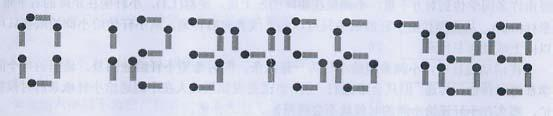/ Vijos / 题库 /

# 描述1. 加号与等号各自需要两根火柴棍

2. 如果A≠B，则A+B=C与B+A=C视为不同的等式（A、B、C>=0）

3. n根火柴棍必须全部用上

# 样例1

## 样例输入1

``````14
``````

## 样例输出1

``````2
``````

# 样例2

## 样例输入2

``````18
``````

## 样例输出2

``````9
``````

1s

# 提示

【输入输出样例1解释】

2个等式为0+1=1和1+0=1。

【输入输出样例2解释】

9个等式为：

0+4=4

0+11=11

1+10=11

2+2=4

2+7=9

4+0=4

7+2=9

10+1=11

11+0=11

NOIP2008提高组第二题。

ID
1496

3

6074

2972

49%

17